Stoichiometry Calculations with Chemical Formulas and Equations Chapter

• Slides: 30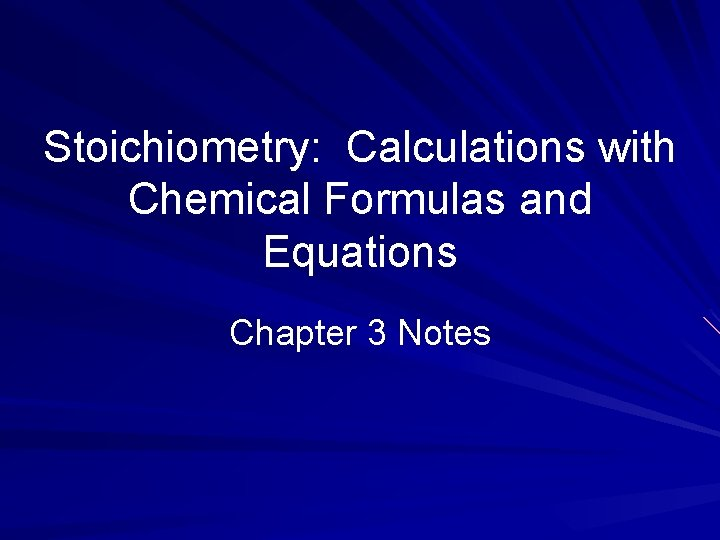Stoichiometry: Calculations with Chemical Formulas and Equations Chapter 3 NotesStoichiometry comes from the Greek words Stoicheion meaning “element” and Metron, meaning “measure” Composition stoichiometry: deals with the mass relationships of elements in compounds Reaction stoichiometry: involves the mass relationships between reactants and products in a chemical reaction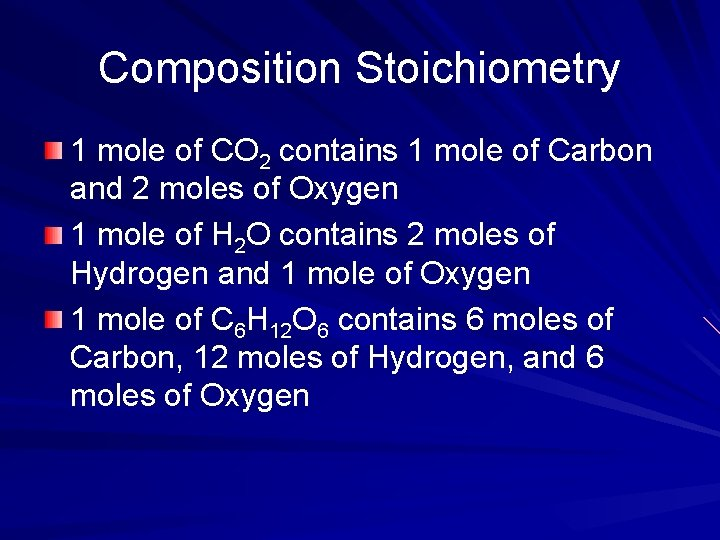Composition Stoichiometry 1 mole of CO 2 contains 1 mole of Carbon and 2 moles of Oxygen 1 mole of H 2 O contains 2 moles of Hydrogen and 1 mole of Oxygen 1 mole of C 6 H 12 O 6 contains 6 moles of Carbon, 12 moles of Hydrogen, and 6 moles of OxygenReaction Stoichiometry Because you can never measure moles directly, you are generally required to calculate the amount in moles from mass which can be measured in the lab The general plan for solving mass-mass problems: Convert mass into moles → multiply by mole ratio → multiply by molar mass of unknown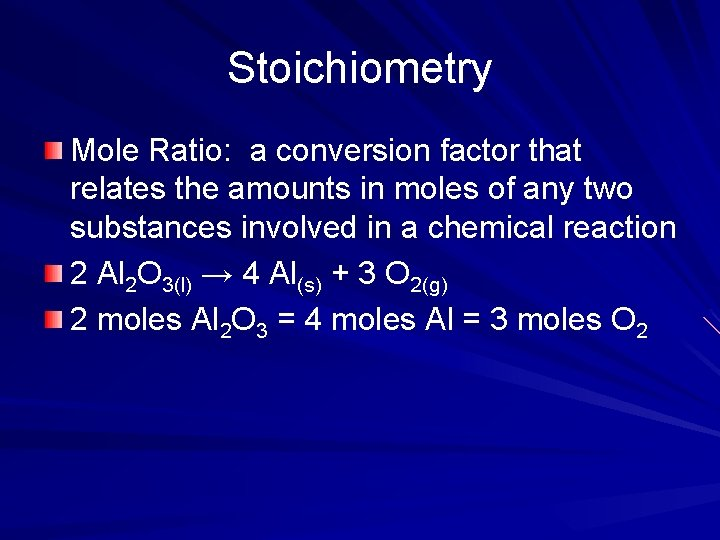Stoichiometry Mole Ratio: a conversion factor that relates the amounts in moles of any two substances involved in a chemical reaction 2 Al 2 O 3(l) → 4 Al(s) + 3 O 2(g) 2 moles Al 2 O 3 = 4 moles Al = 3 moles O 2Sample Problem Laughing gas (N 2 O) is sometimes used as an anesthetic in dentistry. It is produced when ammonium nitrate is decomposed to form N 2 O and water. How many grams of ammonium nitrate are required to produce 33. 0 g of N 2 O? How many grams of water are produced in this reaction?Reaction Stoichiometry Combustion analysis: when a compound containing carbon and hydrogen is combusted completely, all the carbon is converted to CO 2, and all the hydrogen is converted to H 2 O The gases are trapped by absorbers which measure the masses This information can be used to calculate the empirical formula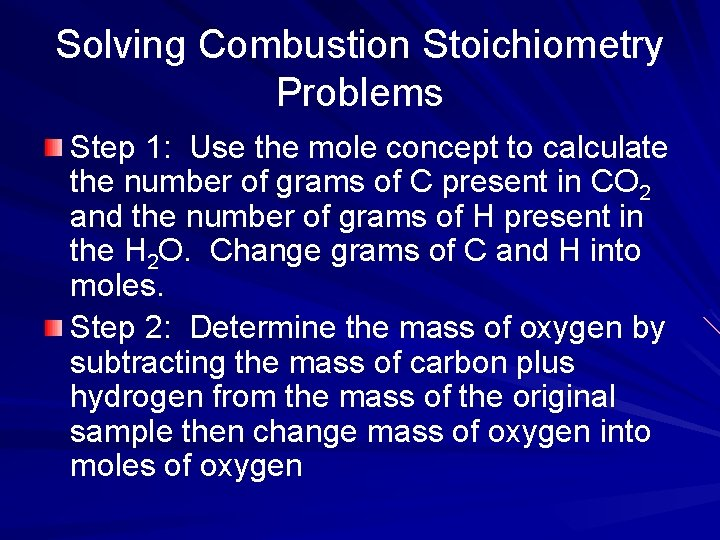Solving Combustion Stoichiometry Problems Step 1: Use the mole concept to calculate the number of grams of C present in CO 2 and the number of grams of H present in the H 2 O. Change grams of C and H into moles. Step 2: Determine the mass of oxygen by subtracting the mass of carbon plus hydrogen from the mass of the original sample then change mass of oxygen into moles of oxygenSolving Combustion Stoichiometry Problems Step 3: Assign the correct number of moles to each atom Step 4: Divide the moles of each element by the smallest mole number. This will give you the empirical formula. Step 5: If given the molecular mass, determine the molecular formula.Sample Problems Isopropyl alcohol is composed of C, H, and O. Combustion of 0. 255 g of isopropyl alcohol produces 0. 561 g CO 2 and 0. 306 g of H 2 O. Determine the empirical formula of isopropyl alcohol. Caproic acid, which is responsible for the foul odor of dirty socks, is composed of C, H, and O atoms. Combustion of a 0. 225 g sample produces 0. 512 g CO 2 and 0. 209 g H 20. What is the empirical formula of Caproic acid?Solving for Unknown Hydrated Crystals Step 1: Find the mass of water evolved during dehydration Step 2: Change the mass of water into moles of water Step 3: Determine the number of moles of anhydrous compound Step 4: Use the ratio of moles of water to moles of anhydrous compound to find the number of water molecules presentLimiting Reactants Suppose Mikayla has to make cheese sandwiches for guests at a party. She has 10 slices of bread and 7 slices of cheese. How many sandwiches can she make? Write an equation that represents the formation of a cheese sandwich. Bd = bread and Ch = cheese Which reactant will run out first? How much of the other reactant will be left?Limiting Reactants In chemical reactions, when one of the reactants is used up the reaction stops, leaving an excess of the other(s). Suppose we have 10 moles of H 2 and 7 moles of O 2. Which one will run out first in the reaction of hydrogen combining with oxygen to form water.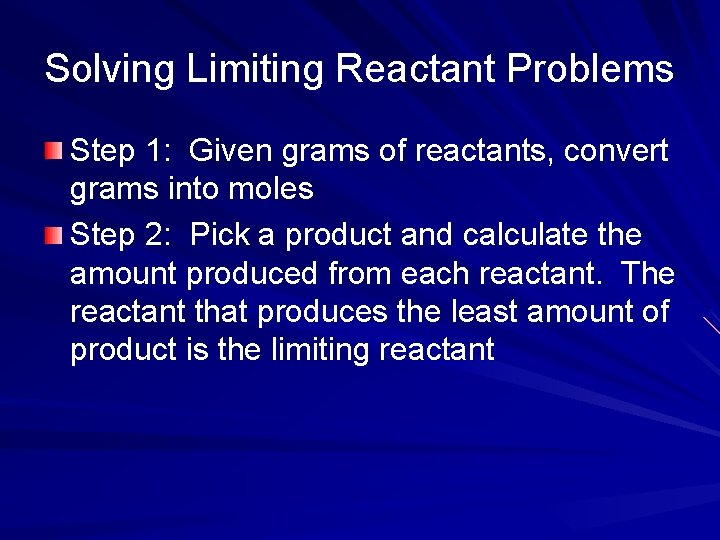Solving Limiting Reactant Problems Step 1: Given grams of reactants, convert grams into moles Step 2: Pick a product and calculate the amount produced from each reactant. The reactant that produces the least amount of product is the limiting reactant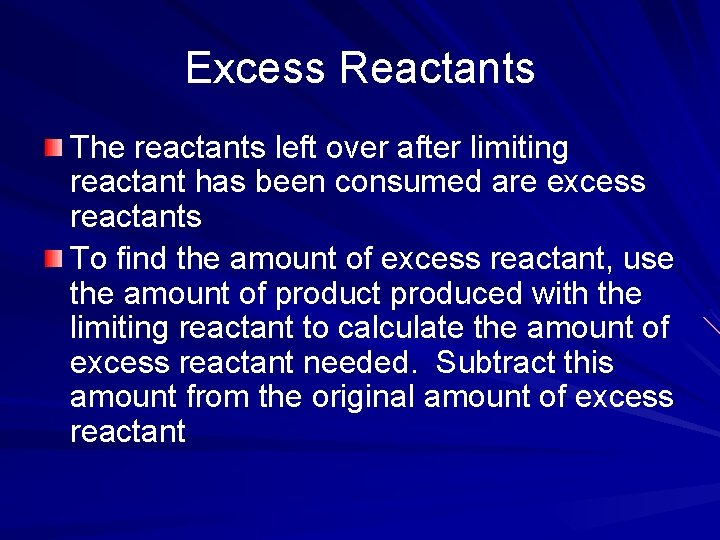Excess Reactants The reactants left over after limiting reactant has been consumed are excess reactants To find the amount of excess reactant, use the amount of product produced with the limiting reactant to calculate the amount of excess reactant needed. Subtract this amount from the original amount of excess reactantTheoretical and Percent Yield Theoretical yield: the amount of product produced from the complete reaction of all the limiting reactant Actual yield: the actual amount of product obtained in a chemical reaction Percent yield: calculated by dividing the actual yield by theoretical yield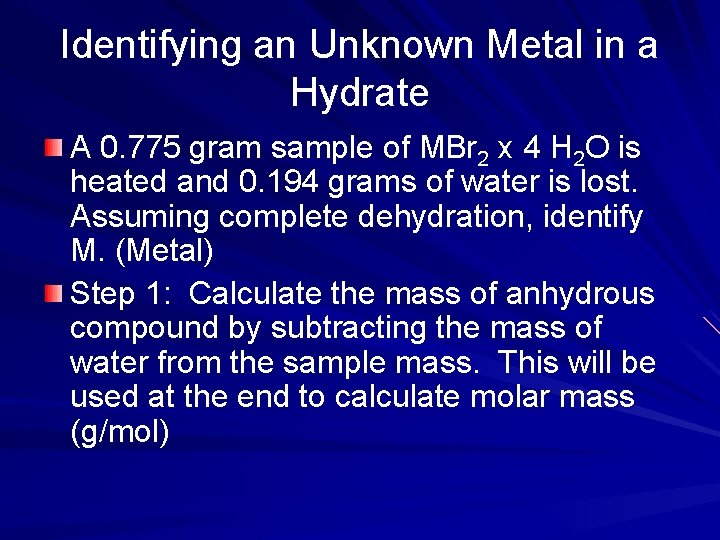Identifying an Unknown Metal in a Hydrate A 0. 775 gram sample of MBr 2 x 4 H 2 O is heated and 0. 194 grams of water is lost. Assuming complete dehydration, identify M. (Metal) Step 1: Calculate the mass of anhydrous compound by subtracting the mass of water from the sample mass. This will be used at the end to calculate molar mass (g/mol)Identifying an Unknown Metal in a Hydrate Step 2: Convert grams of water lost to moles of anhydrous compound using the mole ratio 1 mol MBr 2 : 4 mol H 2 O Step 3: Calculate the molar mass of anhydrous compound by dividing the grams of anhydrous compound by the moles of anhydrous compound. Step 4: Subtract the mass of the nonmetal to find the mass of the metal for identification.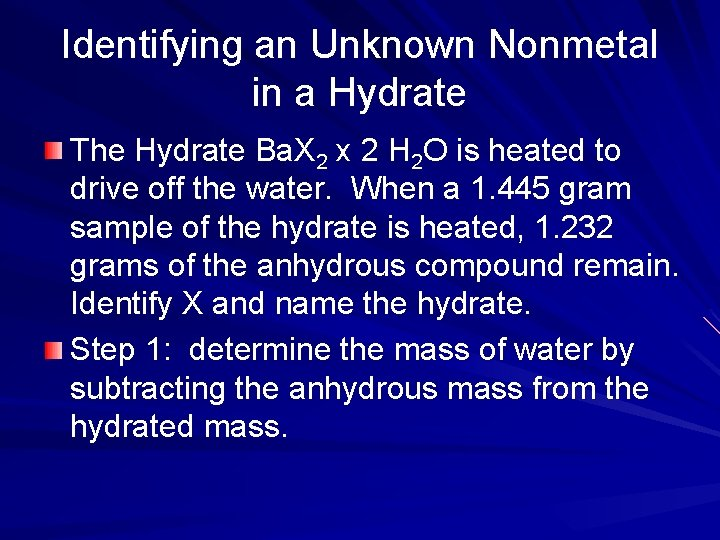Identifying an Unknown Nonmetal in a Hydrate The Hydrate Ba. X 2 x 2 H 2 O is heated to drive off the water. When a 1. 445 gram sample of the hydrate is heated, 1. 232 grams of the anhydrous compound remain. Identify X and name the hydrate. Step 1: determine the mass of water by subtracting the anhydrous mass from the hydrated mass.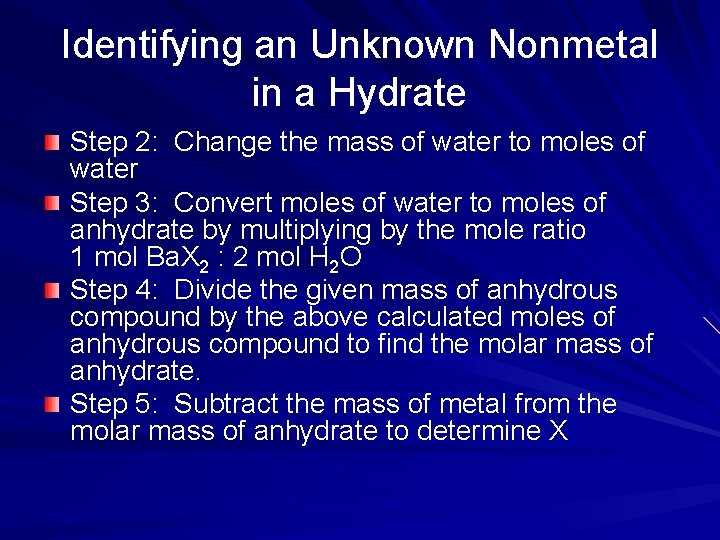Identifying an Unknown Nonmetal in a Hydrate Step 2: Change the mass of water to moles of water Step 3: Convert moles of water to moles of anhydrate by multiplying by the mole ratio 1 mol Ba. X 2 : 2 mol H 2 O Step 4: Divide the given mass of anhydrous compound by the above calculated moles of anhydrous compound to find the molar mass of anhydrate. Step 5: Subtract the mass of metal from the molar mass of anhydrate to determine X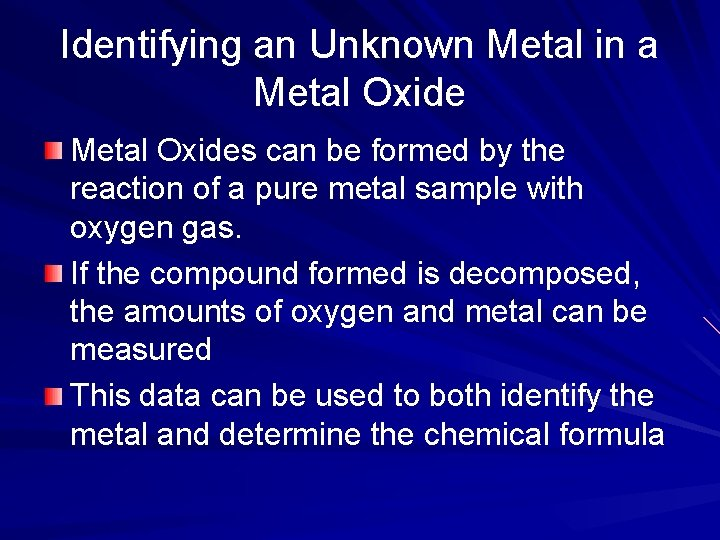Identifying an Unknown Metal in a Metal Oxides can be formed by the reaction of a pure metal sample with oxygen gas. If the compound formed is decomposed, the amounts of oxygen and metal can be measured This data can be used to both identify the metal and determine the chemical formulaIdentifying an Unknown Metal in a Metal Oxide A sample of 1. 443 g of an unknown metal reacts with oxygen to form 1. 683 g of M 2 O 3 Write the balanced equation Find the molar mass of M Name M 2 O 3 Step 1: Find the grams of oxygen by subtracting the given mass of the metal from the given mass of the metal oxideIdentifying an Unknown Metal in a Metal Oxide Step 2: convert grams of oxygen into moles of oxygen Step 3: multiply the moles of oxygen by the mole ratio relating metal to oxygen in the metal oxide to find the moles of metal Step 4: calculate molar mass (g/mol) by dividing the given grams of metal by the calculated moles of metal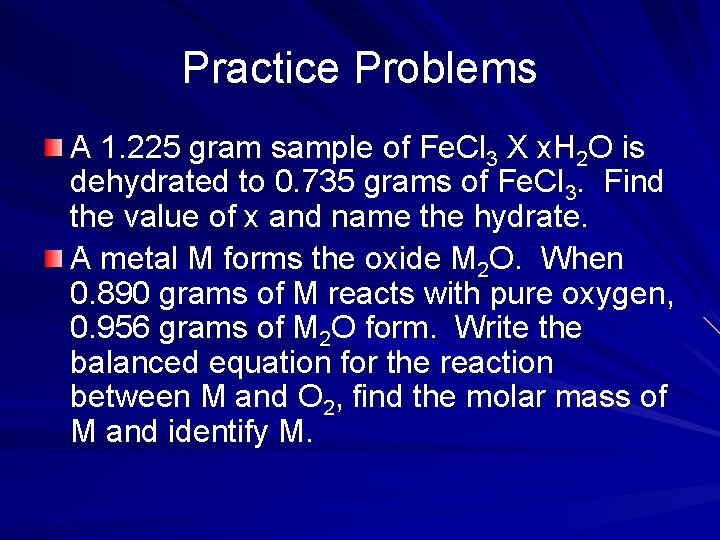Practice Problems A 1. 225 gram sample of Fe. Cl 3 X x. H 2 O is dehydrated to 0. 735 grams of Fe. Cl 3. Find the value of x and name the hydrate. A metal M forms the oxide M 2 O. When 0. 890 grams of M reacts with pure oxygen, 0. 956 grams of M 2 O form. Write the balanced equation for the reaction between M and O 2, find the molar mass of M and identify M.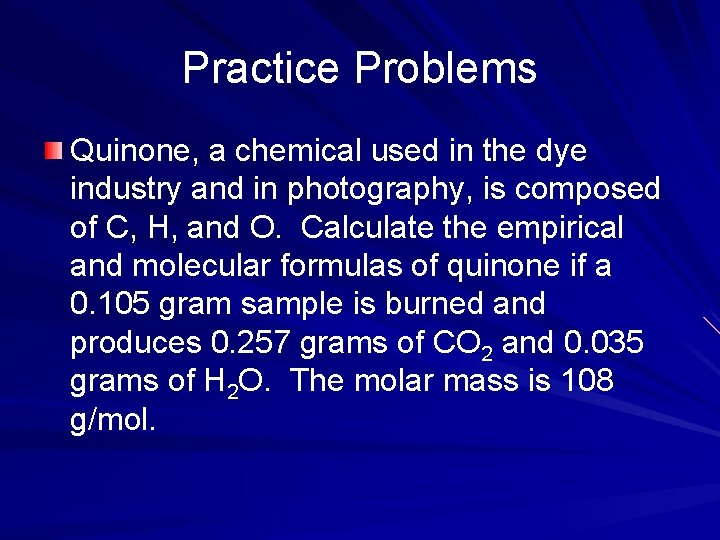Practice Problems Quinone, a chemical used in the dye industry and in photography, is composed of C, H, and O. Calculate the empirical and molecular formulas of quinone if a 0. 105 gram sample is burned and produces 0. 257 grams of CO 2 and 0. 035 grams of H 2 O. The molar mass is 108 g/mol.Practice Problems The analysis of an unknown metal chloride, MCl 3, shows that it contains 67. 2% Cl by mass. Calculate the atomic mass of M and identify the element. Calculate the atomic weight of the metal (M) that forms a compound with the formula MCl 2 that is 74. 5% Cl by mass.Practice Problems A sample of 5. 000 g of Cr(NO 3)3 X x. H 2 O is heated to dryness. A mass of 2. 975 g anhydrous Cr(NO 3)3 remains. Calculate x and name the hydrate. This metal cation requires a Roman numeral.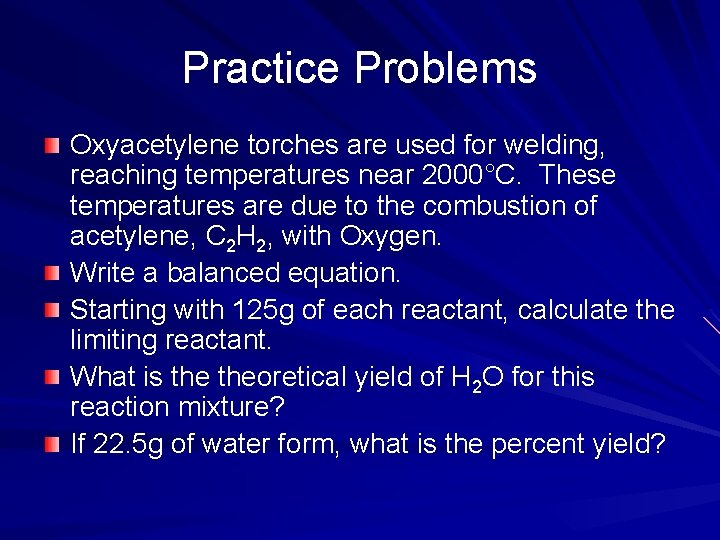Practice Problems Oxyacetylene torches are used for welding, reaching temperatures near 2000°C. These temperatures are due to the combustion of acetylene, C 2 H 2, with Oxygen. Write a balanced equation. Starting with 125 g of each reactant, calculate the limiting reactant. What is theoretical yield of H 2 O for this reaction mixture? If 22. 5 g of water form, what is the percent yield?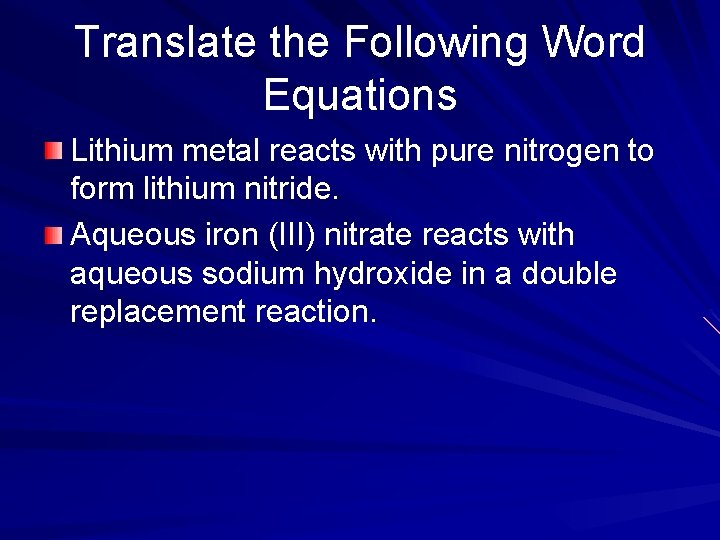Translate the Following Word Equations Lithium metal reacts with pure nitrogen to form lithium nitride. Aqueous iron (III) nitrate reacts with aqueous sodium hydroxide in a double replacement reaction.Translate the Following Word Equations and Predict Products Carbon dioxide reacts with water (synthesis). Zinc metal reacts with copper (II) sulfate (single replacement). Aqueous barium nitrate reacts with aqueous sodium sulfate (double replacement).